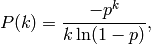# numpy.random.logseries¶

numpy.random.logseries(p, size=None)

Draw samples from a Logarithmic Series distribution.

Samples are drawn from a Log Series distribution with specified parameter, p (probability, 0 < p < 1).

Parameters : loc : float scale : float > 0. size : {tuple, int} Output shape. If the given shape is, e.g., (m, n, k), then m * n * k samples are drawn. samples : {ndarray, scalar} where the values are all integers in [0, n].

scipy.stats.distributions.logser
probability density function, distribution or cumulative density function, etc.

Notes

The probability density for the Log Series distribution iswhere p = probability.

The Log Series distribution is frequently used to represent species richness and occurrence, first proposed by Fisher, Corbet, and Williams in 1943 . It may also be used to model the numbers of occupants seen in cars .

References

 [R88] Buzas, Martin A.; Culver, Stephen J., Understanding regional species diversity through the log series distribution of occurrences: BIODIVERSITY RESEARCH Diversity & Distributions, Volume 5, Number 5, September 1999 , pp. 187-195(9).
 [R89] Fisher, R.A,, A.S. Corbet, and C.B. Williams. 1943. The relation between the number of species and the number of individuals in a random sample of an animal population. Journal of Animal Ecology, 12:42-58.
 [R90] D. J. Hand, F. Daly, D. Lunn, E. Ostrowski, A Handbook of Small Data Sets, CRC Press, 1994.
 [R91] Wikipedia, “Logarithmic-distribution”, http://en.wikipedia.org/wiki/Logarithmic-distribution

Examples

Draw samples from the distribution:

>>> a = .6
>>> s = np.random.logseries(a, 10000)
>>> count, bins, ignored = plt.hist(s)


# plot against distribution

>>> def logseries(k, p):
...     return -p**k/(k*log(1-p))
>>> plt.plot(bins, logseries(bins, a)*count.max()/
logseries(bins, a).max(), 'r')
>>> plt.show()


#### Previous topic

numpy.random.lognormal

#### Next topic

numpy.random.multinomial